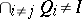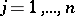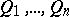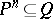# Primary decomposition

(diff) ← Older revision | Latest revision (diff) | Newer revision → (diff)
A representation of an idealof a ring(or of a submoduleof a module) as an intersection of primary ideals (primary submodules, cf. Primary ideal). The primary decomposition generalizes the factorization of an integer into a product of powers of distinct prime numbers. The existence of primary decompositions in a polynomial ring was proved by E. Lasker , and in an arbitrary commutative Noetherian ring by E. Noether . Letbe a commutative Noetherian ring. A primary decompositionis called irreducible iffor anyand if the radicalsof the idealsare pairwise distinct (the radical of a primary idealis the unique prime idealsuch thatfor some natural number). The set of prime idealsis uniquely determined by the ideal(the first uniqueness theorem for primary decompositions). The minimal elements (with respect to inclusion) of this set are called the isolated prime ideals of, the other elements are called the imbedded prime ideals. The primary ideals corresponding to isolated prime ideals are also uniquely determined by(the second uniqueness theorem for primary decompositions, cf. ). The isolated prime ideals of an idealof a polynomial ring over a field correspond to the irreducible components of the affine variety of roots of. There are various generalizations of the notion of primary decomposition. The axiomatization of primary decompositions led to the development of the additive theory of ideals.